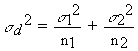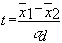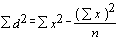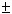## This site is no longer maintained and has been left for archival purposes

### Text and links may be out of date

T-TEST

Student's t-test

We use this test for comparing the means of two samples (or treatments), even if they have different numbers of replicates. In simple terms, the t-test compares the actual difference between two means in relation to the variation in the data (expressed as the standard deviation of the difference between the means).

Procedure

First, we will see how to do this test using "pencil and paper" (with a calculator to help with the calculations). Then we can see how the same test can be done in a spreadsheet package (Microsoft 'Excel')

1. We need to construct a null hypothesis - an expectation - which the experiment was designed to test. For example:

• If we are analysing the heights of pine trees growing in two different locations, a suitable null hypothesis would be that there is no difference in height between the two locations. The student's t-test will tell us if the data are consistent with this or depart significantly from this expectation. [NB: the null hypothesis is simply something to test against. We might well expect a difference between trees growing in a cold, windy location and those in a warm, protected location, but it would be difficult to predict the scale of that difference - twice as high? three times as high? So it is sensible to have a null hypothesis of "no difference" and then to see if the data depart from this.

2. List the data for sample (or treatment) 1.

3. List the data for sample (or treatment) 2.

4. Record the number (n) of replicates for each sample (the number of replicates for sample 1 being termed n1 and the number for sample 2 being termed n2)

5. Calculate mean of each sample (1 and2).

6. Calculate s 2 for each sample; call these s 12 and s 22 [Note that actually we are using S2 as an estimate of s 2 in each case]

5. Calculate the variance of the difference between the two means (sd2) as follows6. Calculate sd (the square root of sd2)

7. Calculate the t value as follows:(when doing this, transpose1 and2 if2 >1 so that you always get a positive value)

8. Enter the t-table at (n1 + n2 -2) degrees of freedom; choose the level of significance required (normally p = 0.05) and read the tabulated t value.

9. If the calculated t value exceeds the tabulated value we say that the means are significantly different at that level of probability.

10. A significant difference at p = 0.05 means that if the null hypothesis were correct (i.e. the samples or treatments do not differ) then we would expect to get a t value as great as this on less than 5% of occasions. So we can be reasonably confident that the samples/treatments do differ from one another, but we still have nearly a 5% chance of being wrong in reaching this conclusion.

Now compare your calculated t value with tabulated values for higher levels of significance (e.g. p = 0.01). These levels tell us the probability of our conclusion being correct. For example, if our calculated t value exceeds the tabulated value for p = 0.01, then there is a 99% chance of the means being significantly different (and a 99.9% chance if the calculated t value exceeds the tabulated value for p = 0.001). By convention, we say that a difference between means at the 95% level is "significant", a difference at 99% level is "highly significant" and a difference at 99.9% level is "very highly significant".

What does this mean in "real" terms? Statistical tests allow us to make statements with a degree of precision, but cannot actually prove or disprove anything. A significant result at the 95% probability level tells us that our data are good enough to support a conclusion with 95% confidence (but there is a 1 in 20 chance of being wrong). In biological work we accept this level of significance as being reasonable.

Student's t-test: a worked example

Suppose that we measured the biomass (milligrams) produced by bacterium A and bacterium B, in shake flasks containing glucose as substrate. We had 4 replicate flasks of each bacterium.

 Bacterium A Bacterium B Replicate 1 520 230 Replicate 2 460 270 Replicate 3 500 250 Replicate 4 470 280 S x 1950 1030 Total (= sum of the 4 replicate values) n 4 4487.5 257.5 Mean (= total / n) S x2 952900 266700 Sum of the squares of each replicate value (S x)2 3802500 1060900 Square of the total (S x). It is not the same as Sx2950625 265225 Sd2 2275 1475s 2 758.3 491.7 s 2 = Sd2 / (n-1)= 189.6 + 122.9 = 312.5 sd2 is the variance of the difference between the means sd = 17.68 = Ö sd2 (the standard deviation of the difference between the means)= 230/17.68 = 13.0

Entering a t table at 6 degrees of freedom (3 for n1 + 3 for n2) we find a tabulated t value of 2.45 (p = 0.05) going up to a tabulated value of 5.96 (p = 0.001). Our calculated t value exceeds these, so the difference between our means is very highly significant. Clearly, bacterium A produces significantly more biomass when grown on glucose than does bacterium B.

[Note that all the time-consuming calculations above can be done on a calculator with memory and statistics functions. Guidance on this can be found in your calculator's instruction booklet. Note also that this test and others can be run on computer packages. Below is a print-out from a package in Microsoft "Excel"]

Student's t-test: the worked example using "Excel" (Microsoft) spreadsheet

[NB: If you cannot find "Data analysis" on Excel then do into "Help" and find "statistical analysis" in the Help index. Different versions of Excel have slightly different commands, so you may not find the following section to be identical to the one you are using.]

The screen for "Excel" (not shown here) has cells arranged in columns A-F... and rows 1-10... For the print-out below, row 1 was used for headings and column A for replicate numbers. The data for Bacterium A were entered in cells B2,3,4,5 and data for Bacterium B in cells C2,3,4,5 of the spreadsheet. From the Tools option at the top of the screen, I selected Data analysis. This displays Analysis options and from the drop-down menu I selected t-test: Two-sample assuming equal variances. Then click OK and enter cells B2-5 for Variable range 1, cells C2-5 for Variable range 2, and a free cell (e.g. A7) for output range (choose the top-left cell of the area where you want the results of the analysis to be displayed). Then click OK and the printout appears.

 Replicate Bacterium A Bacterium B 1 520 230 2 460 270 3 500 250 4 470 280 t-Test: Two-Sample Assuming Equal Variances Bacterium A Bacterium B Mean 487.5 257.5 Variance 758.3333 491.6667 Observations 4 4 Pooled Variance 625 Hypothesized Mean Difference 0 (The test will "ask" what is the probability of obtaining our given results by chance if there is no difference between the population means?) df 6 t Stat 13.01076 (This shows the t value calculated from the data) P(T<=t) one-tail 6.35E-06 t Critical one-tail 1.943181 P(T<=t) two-tail 1.27E-05 (This shows the probability of getting our calculated t value by chance alone. That probability is extremely low, so the means are significantly different) t Critical two-tail 2.446914 (This shows the t value that we would need to exceed in order for the difference between the means to be significant at the 5% level)

We are given the column means, the pooled variance, the number of observations (n), the null hypothesis (that there is no difference between the population means), the degrees of freedom, the calculated t value, and four other entries. The first two of these refer to a one-tailed t-test (i.e. if we wish to test only that one particular mean is larger (or smaller) than the other. The final two entries refer to a two-tailed test, where we do not specify the "direction" of the test. For most purposes, we use a two-tailed test. In each case we are shown the probability that our calculated t-value is equal to or less than the "tabulated" t-value (shown as "Critical t"). Note that the calculated t-value (13.0) vastly exceeds the critical t-value (two-tailed); the means for the bacteria are significantly different at p = 1.27 x 10-5. In other words, there is a probability of about 1 in 100,000 that we would get our observed difference between the means by chance alone.

The analysis for a t-test always pools the variances and, strictly speaking, it is only valid if the variances of the two treatments are similar. In the analysis above we could have selected the option "t-test: Two-sample assuming unequal variances". This would have given us the same result from our particular set of data but would have shown their separate variances as 758.33 for bacterium A and 491.67 for bacterium B.

For interest, let us ask if these variances are different in statistical terms. There is a simple test for this: divide the larger variance by the smaller (758.33 / 491.67 = 1.54) and compare this variance ratio with a value from a table of ‘F’ (variance ratio) for p = 0.05. For 2 treatments there is one degree of freedom between treatments (shown as n1 in the table) and 6 df within treatments (3 for each treatment), shown as n2 in the table. The tabulated F value is 6.0. Our variance ratio (1.54) is less than this, so our variances do not differ significantly. Our t-test was valid.

Student's t-test: deciphering the data in publications

Here are some results taken randomly from a scientific paper. Look at any scientific journal and you will find something similar to this:

Intracellular water volume for Streptococcus mutans; m l (mg dry wt.)-1

 pH 7.0 2.490.06 pH 5.5 2.620.05 (meansSE; n = 6)

Note that the authors give us all the information that we need to test for significance. They tell us that the values are means of 6 replicates for each pH level, with the standard errors of those means.

If we go back to Descriptive Statistics, we will see that a standard error (sn ) for a mean is calculated from the standard deviation (s ) as follows: sn = s / Ö n.

If we square s / Ö n, we get s2 / n.

Now, if we go back to one of the steps in the t-test, we see that we calculate:In this step we add the separate values of s2/n for each mean.

In other words, to do a t test on the published data, all we need do is to square the standard errors. We will do it now:

 pH 7.0 pH 5.5 MeanSE 2.410.06 2.620.05 n 6 6 SE2 (= s2/n) 0.0036 0.0025= 0.0036 + 0.0025 = 0.0061 sd = Ö 0.0061 = 0.0781(2.62 - 2.41)/0.0781 = 2.689 The critical t value (p = 0.05) for 10 degrees of freedom (n1 + n2 - 2) is 2.23. The calculated value exceeds this. There is less than a 5% probability of getting a value as high as this by chance alone. So we can be reasonably confident that pH does affect the intracellular water volume of the bacterium.
 CONTENTS WHAT TEST DO I NEED? STATISTICAL TESTS: Student's t-test for comparing the means of two samples Paired-samples test. (like a t-test, but used when data can be paired) Analysis of variance for comparing means of three or more samples: Chi-squared test for categories of data Poisson distribution for count dataCorrelation coefficient and regression analysis for line fitting: TRANSFORMATION of data: percentages, logarithms, probits and arcsin values

## This site is no longer maintained and has been left for archival purposes

### Text and links may be out of date

Accessibility Statement